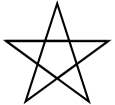# 5.9. Chapter 5 Exercises¶

1. There are 3 syntax errors in the following code. Fix it to work correctly without errors.

2. The code below is correct, but the lines are in the wrong order. Fix it so that it runs properly.

3. The following program is missing things on lines 1, 2, and 3. Add the missing parts.

4. Rearrange the code so it draws a square.

5. The following code has 3 syntax errors. Fix the errors so that the code runs.

6. Fix the 6 errors in the following code.

7. The following code draws two lines of a rectangle. Add code to finish drawing the rectangle.

8. You need to fix or add 4 things so that the code runs.

9. The following code is missing 3 lines that do the required set-up. Add them so that the code runs.

10. Finish the code so that it draws an equilateral triangle.

11. Create a drawing that includes penup, pendown, and pensize.

12. Fix the 5 errors.

13. Create a drawing with at least 3 colors and using at least 3 turtles.

14. Fix the errors.

15. Write code below to draw a diamond shape.

16. Write code that spells CS in block letters (it will look more like C5).

17. Write code below to draw a star like this picture.